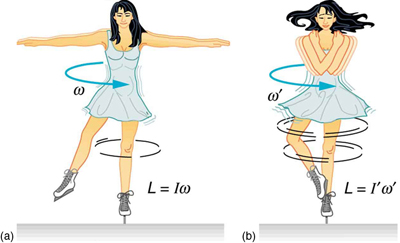# How does moment of inertia affect angular velocity?

Oct 22, 2015

Like this:

#### Explanation:

The expression for angular momentum is:

$L = I \times \omega$

Where $I$ is the moment of inertia and $\omega$ is the angular velocity.

Angular momentum is conserved so if $I$ is decreased you can see from the expression that $\omega$ must increase.

The skater in the graphic has utilised this principle. She brings in her arms to decrease her moment of inertia. As a result her angular velocity has increased i.e she spins faster.